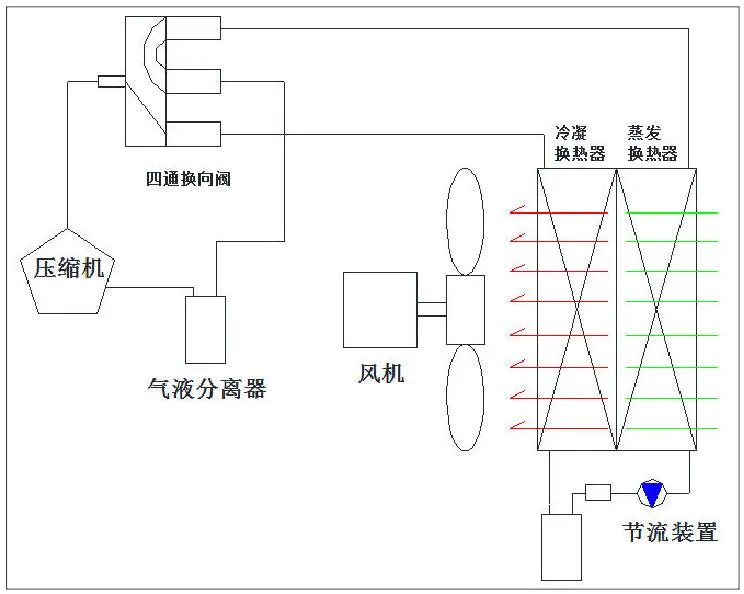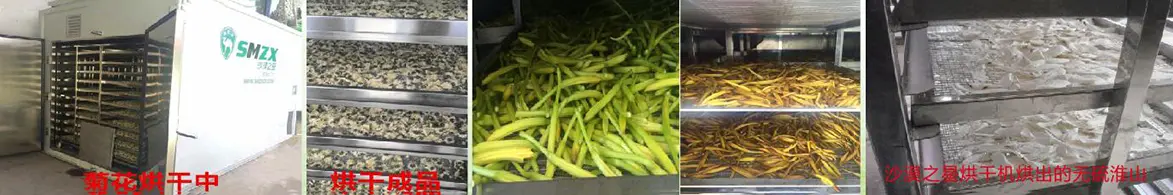•Engineering drying room

• product detailsFigure: Schematic diagram of air drying dehumidifier

To calculate the barn, you need to know three basic data:

A. The amount of raw meal per drying, the unit is KG;

B. The quantity of dry material per drying is K;

The time of each drying, the unit is: hour;

Basic calculations and oven selection;

A. Calculate the amount of dehumidification; according to the raw material amount - dry material amount = dehumidification amount

B. Calculate the hourly dehumidification amount according to the time required by the other party; that is, the dehumidification amount/drying time obtained by the above "A" = the average dehumidification amount per hour

C. Compare our ovens according to the calculated hourly dehumidification capacity. Choose the oven's dehumidification capacity to be slightly larger than the calculated "hourly dehumidification amount".3